## Welcome and Introduction

What is regression?

regression: predict a numerical outcome (“dependent variable”) from a set of inputs (“independent variables”).

• statistical sense: predicting the expected value of the outcome.

• casual sense: predicting a numerical outcome, rather than a discrete one.

• how many units will we sell? (Regression)

• will this customer buy our product (yes/no)? (Classification)

• what price will the customer pay for our product? (Regression)

Regression from a machine learning perspective

• scientific mindset: modeling to understand the data generation process
• engineering mindset: modeling to predict accurately

machine learning: engineering mindset

## Linear regression - the fundamental method

Linear regression

$$y = \beta_0 + \beta_1x_1 + \beta_2x_2 + \ldots$$

• $$y$$ is linearly related to each $$x_i$$
• each $$x_i$$ contributes additively to $$y$$

Linear regression in R: lm()

cmodel <- lm(temperature ~ chirps_per_sec, data = cricket)
• formula: temperature ~ chirps_per_sec
• data frame: cricket

formulas

• LHS: outcome
• RHS: inputs
• use + for multiple inputs

fmla_1 <- as.formula("temperature ~ chirps_per_sec")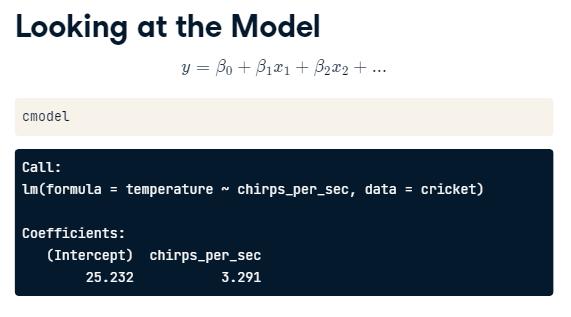For every unit increase in chirp rate, temperature should increase 3.291 degrees, everything else held constant.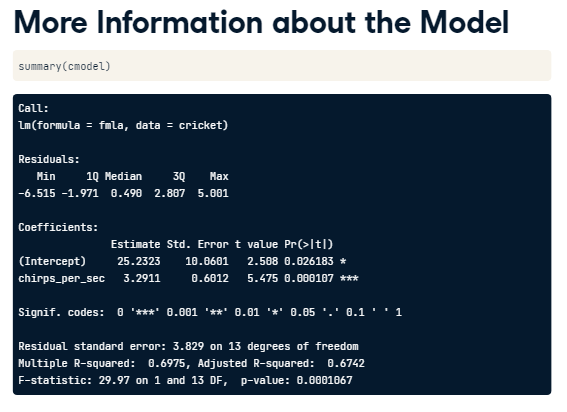broom::glance(cmodel)
sigr::wrapFTest(cmodel)

Code a simple one-variable regression

For the first coding exercise, you’ll create a formula to define a one-variable modeling task, and then fit a linear model to the data. You are given the rates of male and female unemployment in the United States over several years (Source).

The task is to predict the rate of female unemployment from the observed rate of male unemployment. The outcome is female_unemployment, and the input is male_unemployment.

The sign of the variable coefficient tells you whether the outcome increases (+) or decreases (-) as the variable increases.

Recall the calling interface for lm() is:

lm(formula, data = ___)
unemployment <- readRDS("_data/unemployment.rds")

# unemployment is loaded in the workspace
summary(unemployment)
##  male_unemployment female_unemployment
##  Min.   :2.900     Min.   :4.000
##  1st Qu.:4.900     1st Qu.:4.400
##  Median :6.000     Median :5.200
##  Mean   :5.954     Mean   :5.569
##  3rd Qu.:6.700     3rd Qu.:6.100
##  Max.   :9.800     Max.   :7.900
# Define a formula to express female_unemployment as a function of male_unemployment
fmla <- female_unemployment ~ male_unemployment

# Print it
fmla
## female_unemployment ~ male_unemployment
# Use the formula to fit a model: unemployment_model
unemployment_model <-  lm(fmla, data = unemployment)

# Print it
unemployment_model
##
## Call:
## lm(formula = fmla, data = unemployment)
##
## Coefficients:
##       (Intercept)  male_unemployment
##            1.4341             0.6945

Good work. The coefficient for male unemployment is positive, so female unemployment increases as male unemployment does. Linear regression is the most basic of regression approaches. You can think of this course as ways to address its limitations.

Examining a model

Let’s look at the model unemployment_model that you have just created. There are a variety of different ways to examine a model; each way provides different information. We will use summary(), broom::glance(), and sigr::wrapFTest().

library(broom)
library(sigr)

# Print unemployment_model
unemployment_model
##
## Call:
## lm(formula = fmla, data = unemployment)
##
## Coefficients:
##       (Intercept)  male_unemployment
##            1.4341             0.6945
# Call summary() on unemployment_model to get more details
summary(unemployment_model)
##
## Call:
## lm(formula = fmla, data = unemployment)
##
## Residuals:
##      Min       1Q   Median       3Q      Max
## -0.77621 -0.34050 -0.09004  0.27911  1.31254
##
## Coefficients:
##                   Estimate Std. Error t value Pr(>|t|)
## (Intercept)        1.43411    0.60340   2.377   0.0367 *
## male_unemployment  0.69453    0.09767   7.111 1.97e-05 ***
## ---
## Signif. codes:  0 '***' 0.001 '**' 0.01 '*' 0.05 '.' 0.1 ' ' 1
##
## Residual standard error: 0.5803 on 11 degrees of freedom
## Multiple R-squared:  0.8213, Adjusted R-squared:  0.8051
## F-statistic: 50.56 on 1 and 11 DF,  p-value: 1.966e-05
# Call glance() on unemployment_model to see the details in a tidier form
glance(unemployment_model)
## # A tibble: 1 x 12
##   r.squared adj.r.squared sigma statistic p.value    df logLik   AIC   BIC
##       <dbl>         <dbl> <dbl>     <dbl>   <dbl> <dbl>  <dbl> <dbl> <dbl>
## 1     0.821         0.805 0.580      50.6 1.97e-5     1  -10.3  26.6  28.3
## # ... with 3 more variables: deviance <dbl>, df.residual <int>, nobs <int>
# Call wrapFTest() on unemployment_model to see the most relevant details
wrapFTest(unemployment_model)
##  "F Test summary: (R2=0.8213, F(1,11)=50.56, p=1.966e-05)."

Great! There are several different ways to get diagnostics for your model. Use the one that suits your needs or preferences the best.

## Predicting once you fit a model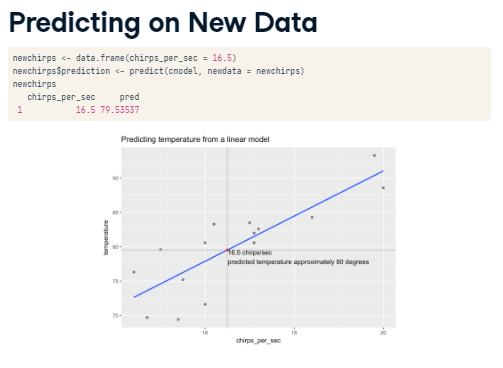Predicting from the unemployment model

In this exercise, you will use your unemployment model unemployment_model to make predictions from the unemployment data, and compare predicted female unemployment rates to the actual observed female unemployment rates on the training data, unemployment. You will also use your model to predict on the new data in newrates, which consists of only one observation, where male unemployment is 5%.

The predict() interface for lm models takes the form

predict(model, newdata)

You will use the ggplot2 package to make the plots, so you will add the prediction column to the unemployment data frame. You will plot outcome versus prediction, and compare them to the line that represents perfect predictions (that is when the outcome is equal to the predicted value).

The ggplot2 command to plot a scatterplot of dframe$outcome versus dframe$pred (pred on the x axis, outcome on the y axis), along with a blue line where outcome == pred is as follows:

ggplot(dframe, aes(x = pred, y = outcome)) +
geom_point() +
geom_abline(color = "blue")
# unemployment is in your workspace
summary(unemployment)
##  male_unemployment female_unemployment
##  Min.   :2.900     Min.   :4.000
##  1st Qu.:4.900     1st Qu.:4.400
##  Median :6.000     Median :5.200
##  Mean   :5.954     Mean   :5.569
##  3rd Qu.:6.700     3rd Qu.:6.100
##  Max.   :9.800     Max.   :7.900
# newrates is in your workspace
newrates <- data.frame(male_unemployment = 5)
newrates
##   male_unemployment
## 1                 5
# Predict female unemployment in the unemployment data set
unemployment$prediction <- predict(unemployment_model) # load the ggplot2 package library(ggplot2) # Make a plot to compare predictions to actual (prediction on x axis) ggplot(unemployment, aes(x = prediction, y = female_unemployment)) + geom_point() + geom_abline(color = "blue")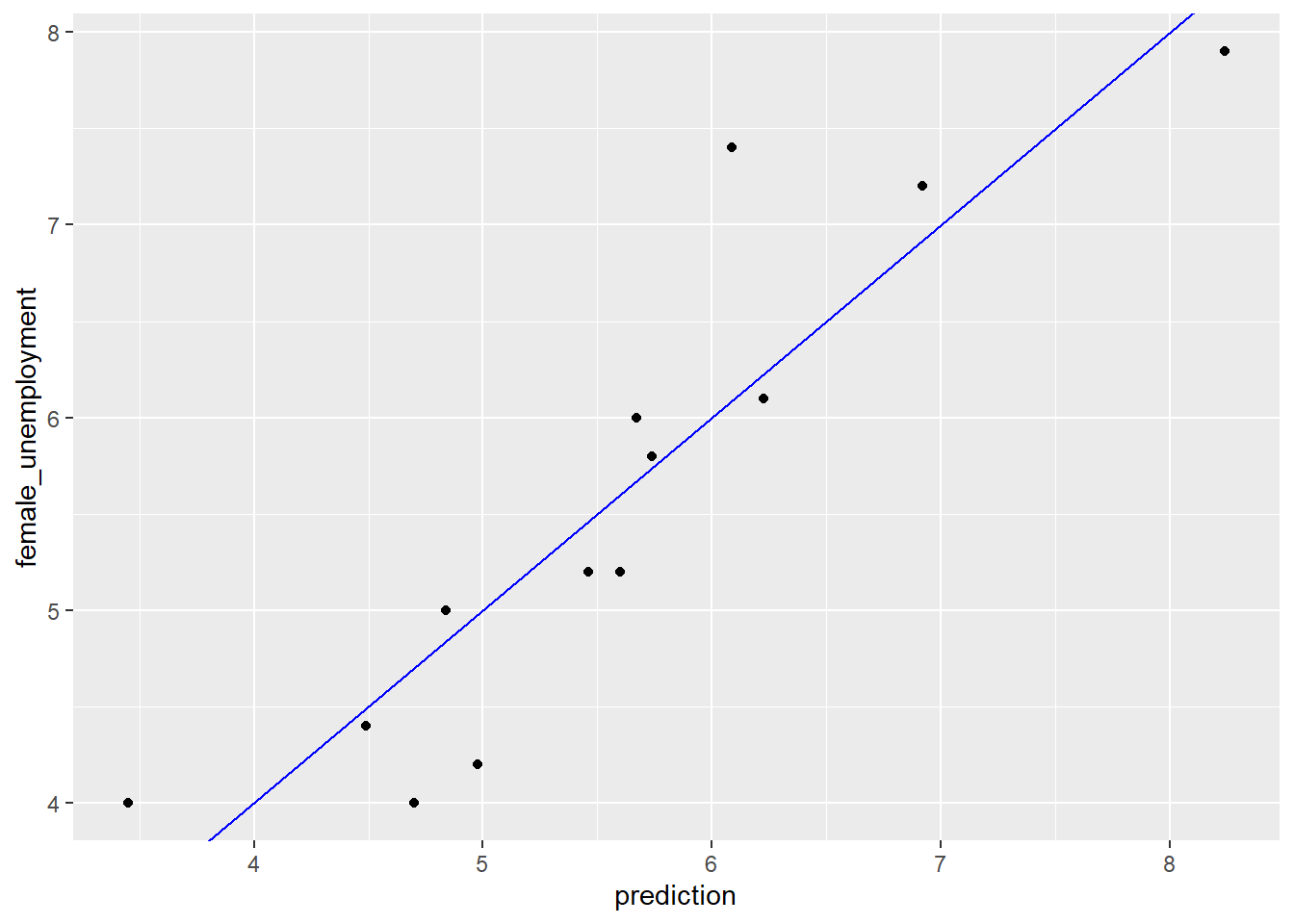# Predict female unemployment rate when male unemployment is 5% pred <- predict(unemployment_model, newdata = newrates) # Print it pred ## 1 ## 4.906757 Good job! While all the modeling algorithms in R implement the predict() method, the call may be a little different for each one. Multivariate linear regression (Part 1) In this exercise, you will work with the blood pressure dataset (Source), and model blood_pressure as a function of weight and age. bloodpressure <- readRDS("_data/bloodpressure.rds") # bloodpressure is in the workspace summary(bloodpressure) ## blood_pressure age weight ## Min. :128.0 Min. :46.00 Min. :167 ## 1st Qu.:140.0 1st Qu.:56.50 1st Qu.:186 ## Median :153.0 Median :64.00 Median :194 ## Mean :150.1 Mean :62.45 Mean :195 ## 3rd Qu.:160.5 3rd Qu.:69.50 3rd Qu.:209 ## Max. :168.0 Max. :74.00 Max. :220 # Create the formula and print it fmla <- blood_pressure ~ age + weight fmla ## blood_pressure ~ age + weight # Fit the model: bloodpressure_model bloodpressure_model <- lm(fmla, data = bloodpressure) # Print bloodpressure_model and call summary() bloodpressure_model ## ## Call: ## lm(formula = fmla, data = bloodpressure) ## ## Coefficients: ## (Intercept) age weight ## 30.9941 0.8614 0.3349 summary(bloodpressure_model) ## ## Call: ## lm(formula = fmla, data = bloodpressure) ## ## Residuals: ## Min 1Q Median 3Q Max ## -3.4640 -1.1949 -0.4078 1.8511 2.6981 ## ## Coefficients: ## Estimate Std. Error t value Pr(>|t|) ## (Intercept) 30.9941 11.9438 2.595 0.03186 * ## age 0.8614 0.2482 3.470 0.00844 ** ## weight 0.3349 0.1307 2.563 0.03351 * ## --- ## Signif. codes: 0 '***' 0.001 '**' 0.01 '*' 0.05 '.' 0.1 ' ' 1 ## ## Residual standard error: 2.318 on 8 degrees of freedom ## Multiple R-squared: 0.9768, Adjusted R-squared: 0.9711 ## F-statistic: 168.8 on 2 and 8 DF, p-value: 2.874e-07 Good! One of the advantages of linear regression is that you can interpret the effects of each variable on the input – to a certain extent. In this case the coefficients for both age and weight are positive, which indicates that blood pressure tends to increase as both age and weight increase. Multivariate linear regression (Part 2) Now you will make predictions using the blood pressure model bloodpressure_model that you fit in the previous exercise. You will also compare the predictions to outcomes graphically. ggplot2 is already loaded in your workspace. Recall the plot command takes the form: ggplot(dframe, aes(x = pred, y = outcome)) + geom_point() + geom_abline(color = "blue") # bloodpressure is in your workspace summary(bloodpressure) ## blood_pressure age weight ## Min. :128.0 Min. :46.00 Min. :167 ## 1st Qu.:140.0 1st Qu.:56.50 1st Qu.:186 ## Median :153.0 Median :64.00 Median :194 ## Mean :150.1 Mean :62.45 Mean :195 ## 3rd Qu.:160.5 3rd Qu.:69.50 3rd Qu.:209 ## Max. :168.0 Max. :74.00 Max. :220 # bloodpressure_model is in your workspace bloodpressure_model ## ## Call: ## lm(formula = fmla, data = bloodpressure) ## ## Coefficients: ## (Intercept) age weight ## 30.9941 0.8614 0.3349 # predict blood pressure using bloodpressure_model :prediction bloodpressure$prediction <- predict(bloodpressure_model)

# plot the results
ggplot(bloodpressure, aes(x = prediction, y = blood_pressure)) +
geom_point() +
geom_abline(color = "blue")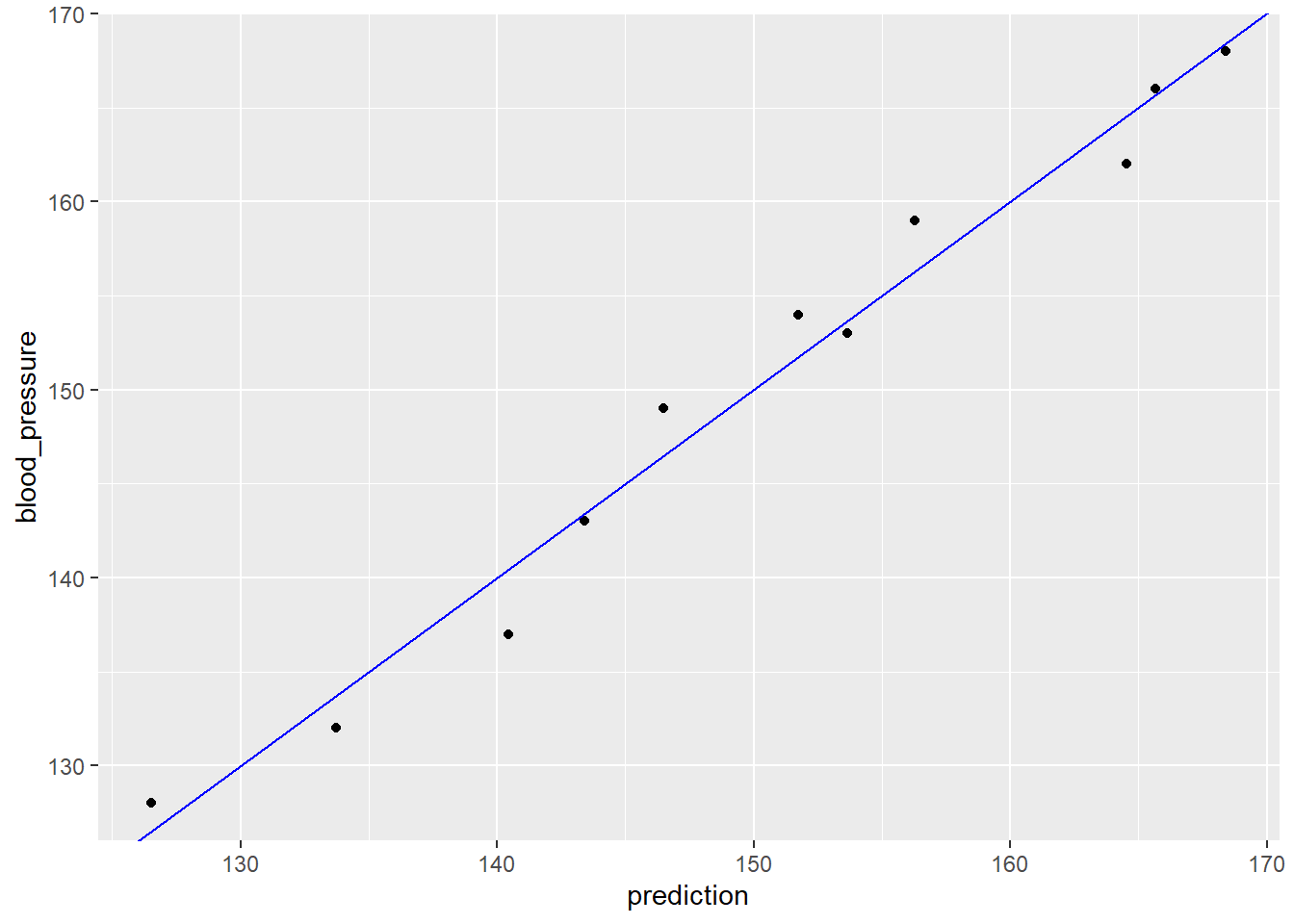Good! The results stay fairly close to the line of perfect prediction, indicating that the model fits the training data well. From a prediction perspective, multivariate linear regression behaves much as simple (one-variable) linear regression does.

## Wrapping up linear regression

Pros and cons of linear regression

• Pros
• easy to fit and apply
• concise
• less prone to overfitting
• interpretable
• Cons
• can only express linear and additive relationships

Collinearity

• when variables are partially correlated
• coefficients may change sign
• high collinearity:
• coefficients (or standard errors) look too large
• model may be unstable

Coming next

• evaluating a regression model
• properly training a model

## Evaluating a model graphically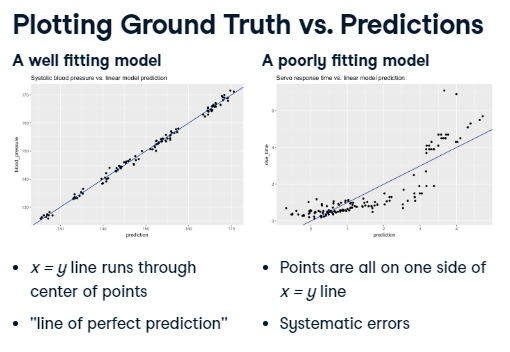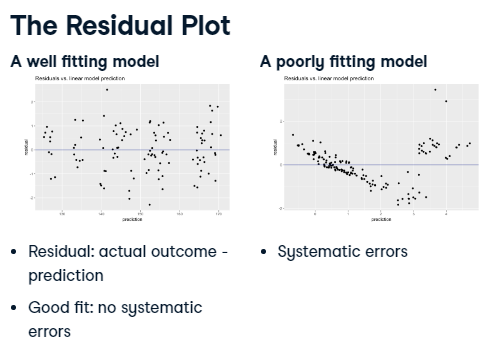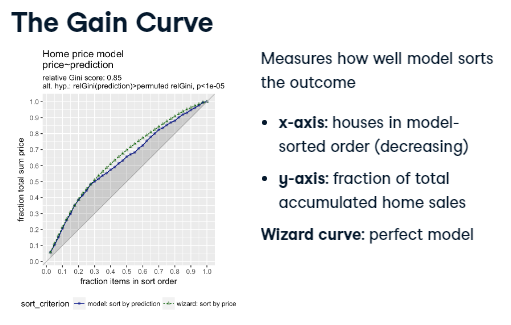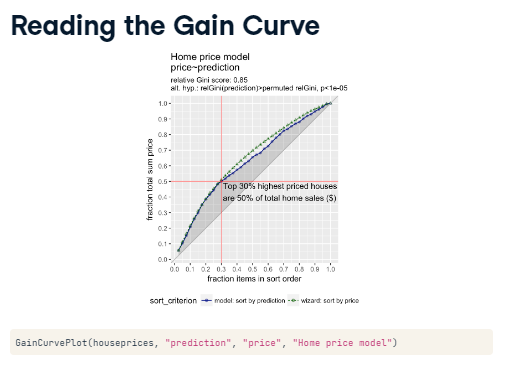Graphically evaluate the unemployment model

In this exercise you will graphically evaluate the unemployment model, unemployment_model, that you fit to the unemployment data in the previous chapter. Recall that the model predicts female_unemployment from male_unemployment.

You will plot the model’s predictions against the actual female_unemployment; recall the command is of the form

ggplot(dframe, aes(x = pred, y = outcome)) +
geom_point() +
geom_abline()

Then you will calculate the residuals:

residuals <- actual outcome - predicted outcome

and plot predictions against residuals. The residual graph will take a slightly different form: you compare the residuals to the horizontal line $$x=0$$ (using geom_hline()) rather than to the line $$x = y$$. The command will be provided.

The data frame unemployment and model unemployment_model are available in the workspace.

# unemployment, unemployment_model are in the workspace
summary(unemployment)
##  male_unemployment female_unemployment   prediction
##  Min.   :2.900     Min.   :4.000       Min.   :3.448
##  1st Qu.:4.900     1st Qu.:4.400       1st Qu.:4.837
##  Median :6.000     Median :5.200       Median :5.601
##  Mean   :5.954     Mean   :5.569       Mean   :5.569
##  3rd Qu.:6.700     3rd Qu.:6.100       3rd Qu.:6.087
##  Max.   :9.800     Max.   :7.900       Max.   :8.240
summary(unemployment_model)
##
## Call:
## lm(formula = fmla, data = unemployment)
##
## Residuals:
##      Min       1Q   Median       3Q      Max
## -0.77621 -0.34050 -0.09004  0.27911  1.31254
##
## Coefficients:
##                   Estimate Std. Error t value Pr(>|t|)
## (Intercept)        1.43411    0.60340   2.377   0.0367 *
## male_unemployment  0.69453    0.09767   7.111 1.97e-05 ***
## ---
## Signif. codes:  0 '***' 0.001 '**' 0.01 '*' 0.05 '.' 0.1 ' ' 1
##
## Residual standard error: 0.5803 on 11 degrees of freedom
## Multiple R-squared:  0.8213, Adjusted R-squared:  0.8051
## F-statistic: 50.56 on 1 and 11 DF,  p-value: 1.966e-05
# Make predictions from the model
unemployment$predictions <- predict(unemployment_model) # Fill in the blanks to plot predictions (on x-axis) versus the female_unemployment rates ggplot(unemployment, aes(x = predictions, y = female_unemployment)) + geom_point() + geom_abline()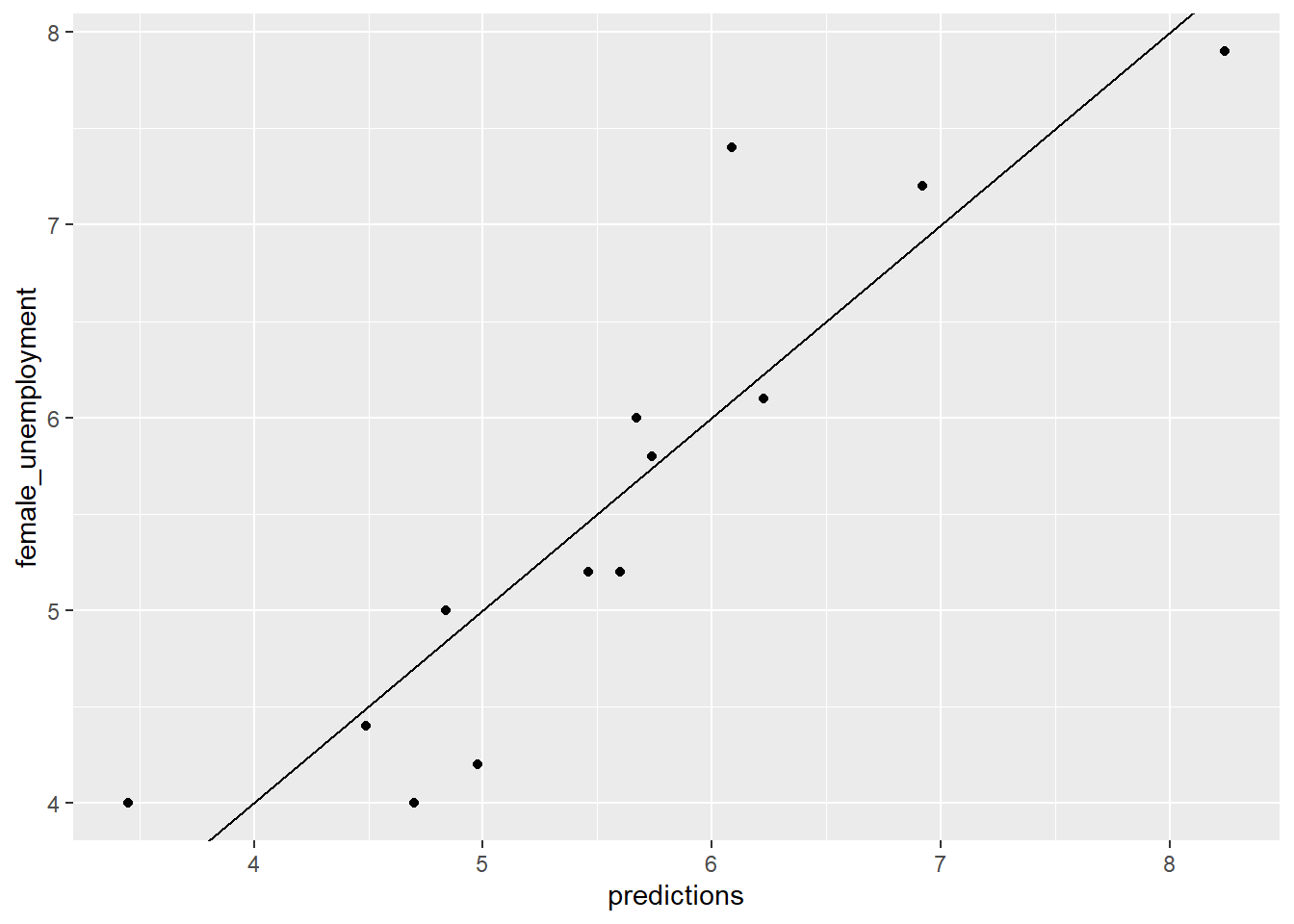# From previous step unemployment$predictions <- predict(unemployment_model)

# Calculate residuals
unemployment$residuals <- unemployment$female_unemployment - unemployment\$predictions

# Fill in the blanks to plot predictions (on x-axis) versus the residuals
ggplot(unemployment, aes(x = predictions, y = residuals)) +
geom_pointrange(aes(ymin = 0, ymax = residuals)) +
geom_hline(yintercept = 0, linetype = 3) +
ggtitle("residuals vs. linear model prediction")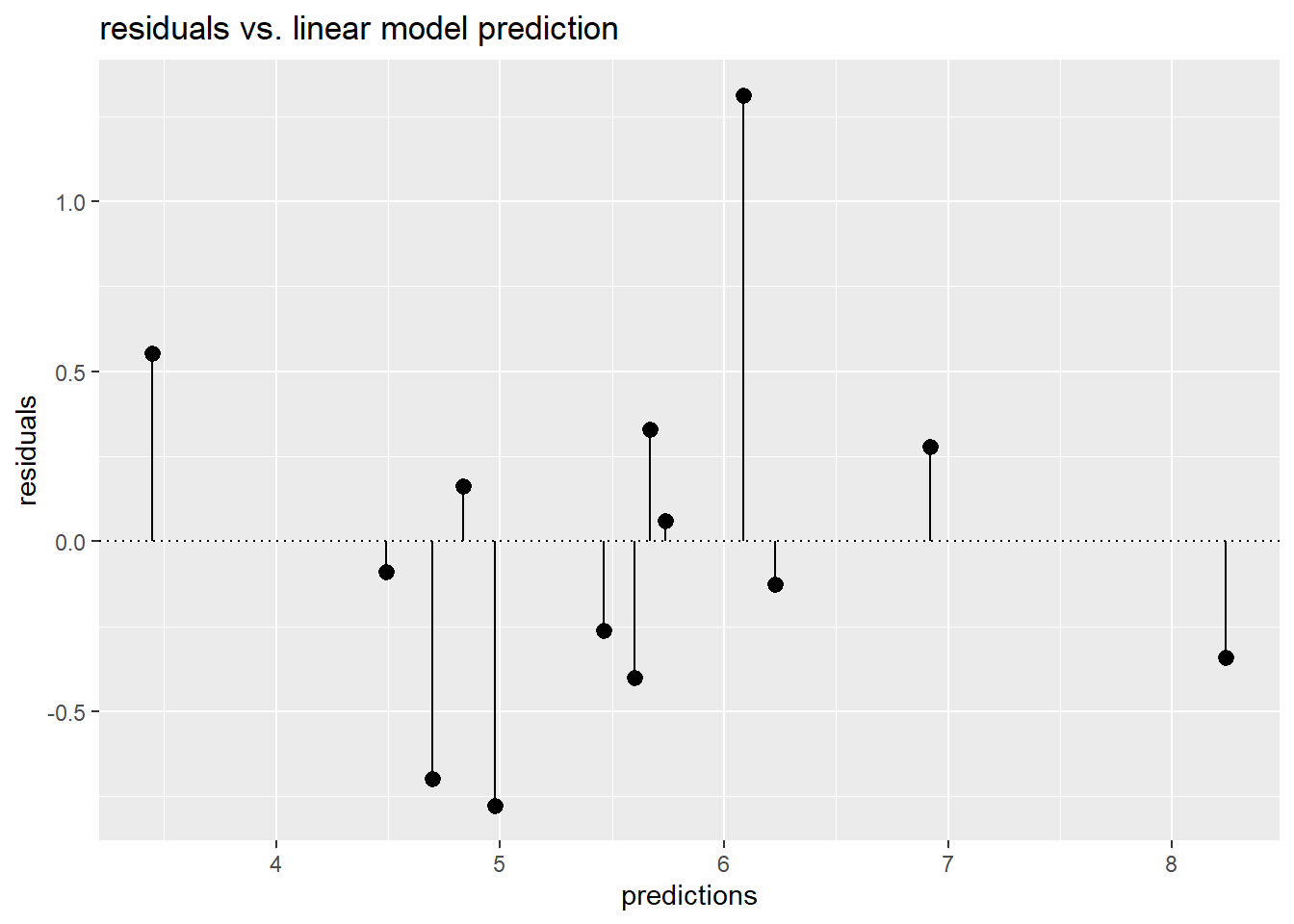Congratulations! You have now evaluated model predictions by comparing them to ground truth, and by examining prediction error.

The gain curve to evaluate the unemployment model

In the previous exercise you made predictions about female_unemployment and visualized the predictions and the residuals. Now, you will also plot the gain curve of the unemployment_model’s predictions against actual female_unemployment using the WVPlots::GainCurvePlot() function.

For situations where order is more important than exact values, the gain curve helps you check if the model’s predictions sort in the same order as the true outcome.

Calls to the function GainCurvePlot() look like:

GainCurvePlot(frame, xvar, truthvar, title)

where

• frame is a data frame
• xvar and truthvar are strings naming the prediction and actual outcome columns of frame
• title is the title of the plot

When the predictions sort in exactly the same order, the relative Gini coefficient is 1. When the model sorts poorly, the relative Gini coefficient is close to zero, or even negative.

# unemployment is in the workspace (with predictions)
summary(unemployment)
##  male_unemployment female_unemployment   prediction     predictions
##  Min.   :2.900     Min.   :4.000       Min.   :3.448   Min.   :3.448
##  1st Qu.:4.900     1st Qu.:4.400       1st Qu.:4.837   1st Qu.:4.837
##  Median :6.000     Median :5.200       Median :5.601   Median :5.601
##  Mean   :5.954     Mean   :5.569       Mean   :5.569   Mean   :5.569
##  3rd Qu.:6.700     3rd Qu.:6.100       3rd Qu.:6.087   3rd Qu.:6.087
##  Max.   :9.800     Max.   :7.900       Max.   :8.240   Max.   :8.240
##    residuals
##  Min.   :-0.77621
##  1st Qu.:-0.34050
##  Median :-0.09004
##  Mean   : 0.00000
##  3rd Qu.: 0.27911
##  Max.   : 1.31254
# unemployment_model is in the workspace
summary(unemployment_model)
##
## Call:
## lm(formula = fmla, data = unemployment)
##
## Residuals:
##      Min       1Q   Median       3Q      Max
## -0.77621 -0.34050 -0.09004  0.27911  1.31254
##
## Coefficients:
##                   Estimate Std. Error t value Pr(>|t|)
## (Intercept)        1.43411    0.60340   2.377   0.0367 *
## male_unemployment  0.69453    0.09767   7.111 1.97e-05 ***
## ---
## Signif. codes:  0 '***' 0.001 '**' 0.01 '*' 0.05 '.' 0.1 ' ' 1
##
## Residual standard error: 0.5803 on 11 degrees of freedom
## Multiple R-squared:  0.8213, Adjusted R-squared:  0.8051
## F-statistic: 50.56 on 1 and 11 DF,  p-value: 1.966e-05
# Load the package WVPlots
library(WVPlots)
## Loading required package: wrapr
# Plot the Gain Curve
GainCurvePlot(unemployment, "predictions", "female_unemployment", "Unemployment model")